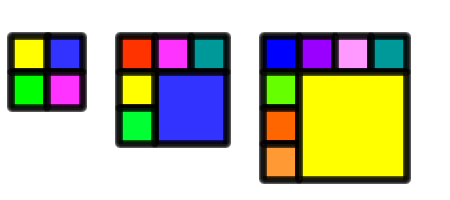#### You may also like### Have You Got It?

Can you explain the strategy for winning this game with any target?### Counting Factors

Is there an efficient way to work out how many factors a large number has?### Is There a Theorem?

Draw a square. A second square of the same size slides around the first always maintaining contact and keeping the same orientation. How far does the dot travel?

# Squares, Squares and More Squares

##### Age 11 to 14Challenge Level

Sarah sent us her answer to this problem:

Once we've got some number of squares, say n, we can get n+3, by subdividing one of the squares into 4. So if we can get 4, 6 and 8 squares, then we can also get 7, 10, 13, ..., 9, 12, 15, ... and 8, 11, 14, ... squares, that is, we can get 4, 6, 7, 8, 9, 10, 11, 12, and in fact everything above this.

Here's how we can get 4, 6, and 8 squares.William noticed that you can't make 2, 3 or 5 squares. Here's his explanation for why not:

For 2 or 3 squares, you'd have to have a small square with at least two of its corners at the corners of the big square. But then it would be the same size as the big square, so we'd only use 1 square. For five squares, you'd have to have a different square in each corner (for the reason just explained). But if you think about it you see that if you're going to have more than four squares, then you actually need at least six, so you can't do it with 5.